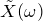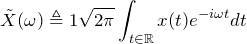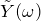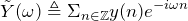# Definitions

July 24, 2019

Before working with Fourier operations, it is best to clearly define the Fourier transforms. In this section, we will primarily focus on two transforms: the Fourier transform and the discrete-time Fourier transform (DTFT).

The Fourier transform operates over continuous functions or signals from the real world before they are sampled by an analog-to-digital converter (ADC). The DTFT works on sequences composed of signals sampled by an ADC.

#### Fourier Transform

The Fourier transform,, of a function x(t) is defined as:

(1)This version of the Fourier transform is also called the unitary Fourier transform.

#### Discrete-Time Fourier Transform

The discrete-time Fourier transform,, of a sequence y(n) is defined as:

(2)Note that while any real-world sequence (e.g. ⦅… , −1, 3, 5, −2, …⦆) has a DTFT, it does not have a Fourier transform.